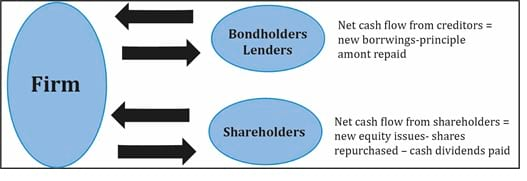Understanding Cash Flow Statements | IFT World
IFT Notes for Level I CFA® Program
IFT Notes for Level I CFA® Program

# Part 3

Investing cash flows

CFI is calculated by examining the change in the gross asset account that results from investing activities. Typically, this change results from purchases or sale of equipment (long term assets). To determine the cash inflow from the sale of equipment, we need to use the expression shown below.

Cash from sale of equipment = historical cost of equipment sold – Accumulated depreciation on equipment sold + Gain on sale of equipment

where:

Historical cost of equipment sold = Beginning balance + equipment purchased – ending equipment
Accumulated depreciation = Beginning balance accumulated depreciation + depreciation expense

Example

The balance sheet extract for Jackal Labs Ltd shows the machinery and accumulated depreciation balances for the years 2011 and 2012.

 2011 2012 Machinery (Gross) $80 million$91 million Accumulated depreciation $25 million$31 million

Further information provided is as follows:

Gain on sale of machinery                           $1.5 million Depreciation expense for 2012$7 million

Capital expenditure on machinery  $14 million What is the cash received from sale of equipment? Solution: Cash from sale of machinery = historical cost of equipment sold – accumulated depreciation on equipment sold + gain on sale of equipment We know the gain is$1.5 million. Calculate the other components in the equation:

Historical cost of equipment sold = beginning balance + equipment purchased – ending balance of equipment = 80 + 14 – 91 = $3 million Accumulated depreciation on equipment sold = beginning value of depreciation + depreciation expense – ending value of depreciation = 25 + 7– 31 =$1 million

Cash from sale of machinery = 3 – 1 + 1.5 = 3.5

Financing cash flows

Cash flow from financing activities refers to cash flows between the firm and the suppliers of capital. Suppliers of capital include creditors, bondholders and shareholders. Similar to investing activities, the presentation of cash flows from financing activities is also identical under both methods. The figure below summarizes the calculation of net cash flows from creditors, bondholders and shareholders.It can be calculated using the following formulae:

(1) CFF = Net cash flow from creditors + Net cash flow from shareholders

(2) Net cash flow from creditors = New borrowings – Principal repaid

(3) Net cash flow from shareholders = New equity issued – Shares repurchased – Cash dividends

Example

The following information is available about company ABC for 2001.

New borrowings                               $10 million Principal repaid$5 million

New equity issued                             $5 million Shares repurchased – Dividends paid$2 million

Calculate CFF.

Solution:

Net cash flow from creditors = New borrowings – Principal repaid = 10 – 5 = $5 million Net cash flow from shareholders = New equity issued – Shares repurchased – Cash dividends = 5 – 0 -2 =$3 million

CFF = Net cash flow from creditors + Net cash flow from shareholders = 5 + 3 = \$8 million

## 15. Conversion of Cash Flows from the Indirect Method to Direct Method

Instructor’s note: The probability of getting tested on this topic on the exam is low.

The operating cash flow from indirect method can be converted to direct by using the three-step process:

• Aggregate all the revenues and expenses.
• Remove all non-cash items from aggregated revenues and expenses and break up remaining items into relevant cash flow items.
• Convert accrual amounts to cash flow amounts by adjusting for changes in corresponding working accounts.

Crash Courses for November CFA Level I, II exams are coming soon!
This is default text for notification bar